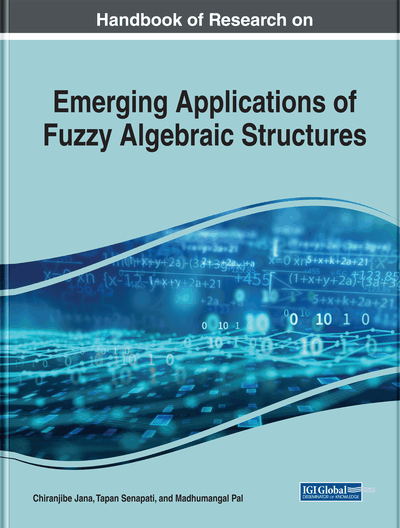Lukaswize Triple-Valued Intuitionistic Fuzzy BCK/BCI-Subalgebras

Chiranjibe Jana (Vidyasagar University, India) and Karping Shum (Yunnan University, China)
DOI: 10.4018/978-1-7998-0190-0.ch012
Available
\$37.50
No Current Special Offers

Abstract

The authors studied the notion of (α,β)-intuitionistic fuzzy BCK/BCI-subalgebras by applying the Lukasiewicz 3-valued implication operator, where α,β∈{∈,q,∈∧q,∈∨q} for α≠∈∧q. In this chapter, an intuitionistic fuzzy set A is an (∈,∈) (or (∈∧q,∈) or (∈,∈∨q))-intuitionistic fuzzy subalgebras if and only if for any p∈(0,1] (or p∈(0,0.5] or p∈(0.5,1]), then Ap is a fuzzy subalgebras of X respectively. Next, the authors defined intuitionistic fuzzy subalgebras with thresholds (s,t) and then provided intuitionistic fuzzy subalgebras with thresholds (0,1) (or (0,0.5) or (0.5,1)) by the concept of quasi-coincidence of fuzzy point respectively. Also, A is an intuitionistic fuzzy subalgebras with thresholds (s,t) if and only if for any p∈(s,t], then cut set A_p is a fuzzy subalgebras.
Chapter Preview
Top

2 Preliminaries

In this section, some elementary aspects that are necessary for this paper are included.

By a BCI.algebra we mean an algebra (X,*,0) of type (2,0) satisfying the following axioms for all x,y,zX.

• (C1) ((x*y)*(x*z))*(z*y)=0

• (C2) (x*(x*y))*y=0

• (C3) x*x=0

• (C4) x*y=0 and y*x=0 imply x=y.

Complete Chapter List

Search this Book:
Reset